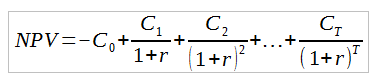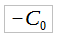# Net Present Value Assignment Help

## Do You Need Help with Your Net Present Value Assignments?

What is the concept behind mergers and acquisitions? At first glance, this may be a complex subject matter to deal with. You will need to understand how to account for the different types of mergers and acquisitions undertaken by entities and know how to compute its corresponding ratios and figures to be reported. Finance Homework can provide you a step-by-step guide on how to effectively and easily understand accounting for mergers and acquisitions.

## What Is Net Present Value (NPV)?

Basically speaking, net present value (NPV) is the net of the present value of all cash inflows and cash outflows of an entity. The reason why we compute for the NPV is to provide a computational analysis on the profitability of a projected investment portfolio or project since it deals with capital budgeting by considering the cost of initial investment and its subsequent inflows – a positive NPV indicates profitability and vice versa.

NPV easily computes the profitability of an investment portfolio using its expected future cash flows and helps determine whether or not it will increase the entity-s value. Another is that it helps the entity see when they could expect to break even or earn profit from the investment portfolio through the analysis of the cash inflows against the initial cost of investment.

The main disadvantage of using NPV in your cost analysis is it does not consider the basic principle of ‘time value of money’, which means that the dollar you earned today will not be valued the same in the future. Additionally, NPV relies heavily on estimations, which imposes risks on the accuracy of the projections made by the entity.

Simple NPV

Using entity estimations in computing for the NPV of an investment project, we can use the formula for simple NPV to determine whether or not the project will be profitable.

The formulas to be used for simple NPV computation are as follow:

`NPV=-Initial Investment+present value of all future annual free cash flows`

or

`NPV=-CO+i=1nCi(1+r)i`

or

`NPV=-CO+C11+r+C2(1+r)2+…+Cn(1+r)n`

Where:

 -CO Initial cost of investment C Cash flow for the period r Discount rate n Time or number of periods

Illustration 1. XYZ Company is deciding whether or not they will push through with Project A, considering that they will need to pay \$500,000 to initiate the project and that the expected annual cash flows, with 5% discount rate, are as follows:

 Year Cash Inflows 1 300,00 2 350,00 3 400,00

Should XYZ Company initiate Project A?

Solution:

```NPV=-CO+C11+r+C2(1+r)2+…+Cn(1+r)n

NPV=-\$500,000+\$300,0001+0.05+\$350,000(1+0.05)2+\$400,000(1+0.05)3

NPV=\$448,709.64```

Yes, XYZ Company should initiate Project A, since its NPV for the three-year projected cash inflows is \$448,709.64.

Discounted cash flow

In order to address the issue on NPV in terms of time value of money, the discounted cash flow (DCF) takes into consideration the fact that the dollar you earned today will be of less value in the future. Basically, it’s still the same in computing for the NPV, only that it discounts it in order to obtain its future value. In DCF, discounting is based on three (3) principles, namely: (1) opportunity; (2) risk; and (3) inflation.

The formula for computing DCF is as follow:

`DCF=C11+r+C2(1+r)2+…+Cn(1+r)n`

or

`Present Value*(1+r)n=Future Value`

Where:

 C Cash flow for the period r Discount rate or weighted average cost of capital (WACC) n Time or number of periods

Illustration 2. Few Tile Company is offered an investment wherein they will receive \$2,000 in three (3) years. How much should Few Tile Company be willing to pay for the said investment? Consider the discount rate of 2.50%.

Solution:

```Present Value*(1+r)n=Future Value

Present Value=Future Value (1+r)n= \$2,000(1+0.025)3

Present Value=\$1,857.20```

Illustration 3. Man Kee Company wants to know the future value of its cash flows, shown below (consider a discount rate of 1.25%):

 Year Cash Flows 1 100,000 2 150,000 3 200,00

Compute for the discounted cash flows of Man Kee Company.

Solution:

```DCF=C11+r+C2(1+r)2+…+Cn(1+r)n= \$100,0001+0.0125+\$150,000(1+0.0125)2+\$200,000(1+0.0125)3

DCF=437,768.26```

## Understanding Net Present Value

The net present value (NPV) of an investment proposal is equal to the present value of its annual free cash flows minus the initial cash outlay. The NPV can be expressed as follows: NPV = – (initial investment) + (present value of all the future annual free cash flows) or= Initial Investment, C= Cash flow,r = discount rate and T = time when any investor takes on a project or investment, it is important to make an estimate of how profitable the venture will be. In the formula, the ­C0 is the initial investment, which is a negative cash flow showing that money is going out as opposed to coming in. Since the money going out is subtracted from the discounted sum of cash flows coming in, the NPV has to be positive in order to be considered a valuable investment. The formula for the discounted sum of all cash flows can be rewritten asThe concepts behind NPV can be difficult to grasp and the equations may appear somewhat intimidating. If you are having difficulties completing a net present value assignment or internal rate of return assignment, don’t panic. Our finance homework service can provide the help you need.

Our service offers net present value assignment assistance. We provide help with any type of finance homework at any academic level including NPV assignments. In finance, it is important to completely understand one concept before moving on to the next as many of them are related. Whether you need some basic help with a homework assignment, assistance with finance test preparation or one-on-one instruction from a tutor who is an expert in the field, we can provide what you need.Well qualified net present value assignment helpThe net present value assignment help you receive will come from well-qualified experts. Our tutors all have degrees in finance or a related field, many of them at the masters and PhD level. They also have extensive experience tutoring finance students and know what is expected at the different academic levels. The finance tutor assigned to you isn’t there just to get you through an assignment. They will ensure you thoroughly understand the concepts and equations involved so that you are able to work NPV problems confidently on your own.
We provide the best finance homework help online. Our finance homework service offers well-qualified finance assistance from experts in the field. There are other benefits of using our service aside from first-rate finance tutoring. These benefits include:

• Guarantees of complete satisfaction and on time delivery with all work we provide
• Budget-friendly rates that students can afford
• Complete customer confidentiality assured